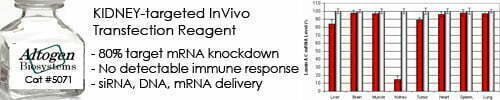••••Shop Products

# DNA Copy Number Calculator

A DNA copy number calculator is a tool that calculates the copy number of a DNA sequence based on the number of reads obtained from a DNA sequencing experiment. The copy number refers to the number of copies of a specific DNA sequence present in a sample, and it can be used to assess the abundance and variability of DNA sequences in various biological samples.

The calculation of DNA copy number involves normalizing the number of reads obtained from a DNA sequencing experiment to the total number of reads and the length of the DNA sequence of interest. This can be done using various normalization methods, such as the RPKM (reads per kilobase per million) or TPM (transcripts per million) methods, which take into account the length of the DNA sequence and the total number of reads obtained from the sequencing experiment.

The formula for calculating DNA copy number using the RPKM method is:

DNA copy number = (number of reads for the DNA sequence / total number of reads) x (10^9 / length of DNA sequence in base pairs)

Where:

• number of reads for the DNA sequence is the number of reads obtained for the specific DNA sequence of interest
• total number of reads is the total number of reads obtained from the DNA sequencing experiment
• length of DNA sequence in base pairs is the length of the DNA sequence of interest in base pairs

To use a DNA copy number calculator, you need to input the values of number of reads for the DNA sequence, total number of reads, and length of DNA sequence, and the calculator will calculate the corresponding DNA copy number for you. The resulting DNA copy number value can be used to assess the abundance and variability of DNA sequences in various biological samples and to compare the expression or presence of different DNA sequences across samples.

DNA copy number calculator:
Enter DNA length:
nt
Enter DNA mass:
μg

Sorry, comments are closed for this post.